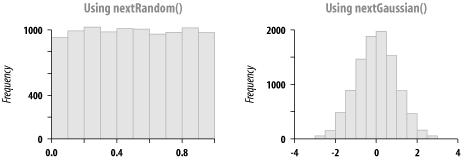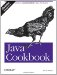# Recipe 5.14 Generating Better Random Numbers

#### Problem

You need to generate better random numbers.

#### Solution

Construct a java.util.Random object (not just any old random object) and call its next*( ) methods. These methods include nextBoolean( ), nextBytes( ) (which fills the given array of bytes with random values), nextDouble( ), nextFloat( ), nextInt( ), and nextLong( ). Don't be confused by the capitalization of Float, Double, etc. They return the primitive types boolean, float, double, etc., not the capitalized wrapper objects. Clear enough? Maybe an example will help:

`// Random2.java  // java.util.Random methods are non-static, so need to construct   Random r = new Random( );  for (int i=0; i<10; i++)     System.out.println("A double from java.util.Random is " + r.nextDouble( ));  for (int i=0; i<10; i++)     System.out.println("An integer from java.util.Random is " + r.nextInt( ));`

You can also use the java.util.Random nextGaussian( ) method, as shown next. The nextDouble( ) methods try to give a "flat" distribution between 0 and 1.0, in which each value has an equal chance of being selected. A Gaussian or normal distribution is a bell-curve of values from negative infinity to positive infinity, with the majority of the values around zero (0.0).

`// Random3.java  Random r = new Random( );  for (int i=0; i<10; i++)      System.out.println("A gaussian random double is " + r.nextGaussian( ));`

To illustrate the different distributions, I generated 10,000 numbers first using nextRandom( ) and then using nextGaussian( ). The code for this is in Random4.java (not shown here) and is a combination of the previous programs with code to print the results into files. I then plotted histograms using the R statistics package (see http://www.r-project.org). The results are shown in Figure 5-1.

##### Figure 5-1. Flat (left) and Gaussian (right) distributionsThe Javadoc documentation for java.util.Random , and the warning in the Introduction about pseudo-randomness versus real randomness.

For cryptographic use, see class java.security.SecureRandom , which provides cryptographically strong pseudo-random number generators (PRNG).Java Cookbook, Second Edition
ISBN: 0596007019
EAN: 2147483647
Year: 2003
Pages: 409
Authors: Ian F Darwin

Similar book on Amazon